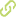# Finite sample properties of virtual reference feedback tuning with two degrees of freedom controllers•
• Overview
•
• Identity
•
• View All
•

### Abstract

• © 2019Here the problem of designing two degrees of freedom controllers for an unknown plant based on input¿output measurements is discussed. Virtual reference feedback tuning aims at minimizing a cost function of the L2-norm type by using a set of data, as no identification process is needed. When constructing this cost function, two model-matching problems are considered between closed loop transfer function and sensitivity function simultaneously. In model-matching procedures, we design virtual input and virtual disturb respectively. Further two filters used to reprocess the input¿output measurements are derived to prove the equivalence between virtual reference feedback tuning and model reference control. After constructing one identification cost without any knowledge of the plant, we derive one bound on the difference between the expected identification cost and its sample identification cost under the condition that the number of data points is finite. Further the correlation property between the input and external noise is considered in deriving this bound. Then we continue to derive one probabilistic bound to quantify this difference through using some probability inequalities and knowledge of control theory. The number of data points is obtained by using generalization of independent block sequence. Finally two simulation examples have been performed to demonstrate the effectiveness of the theories proposed in this paper.

### Publication date

• January 1, 2019